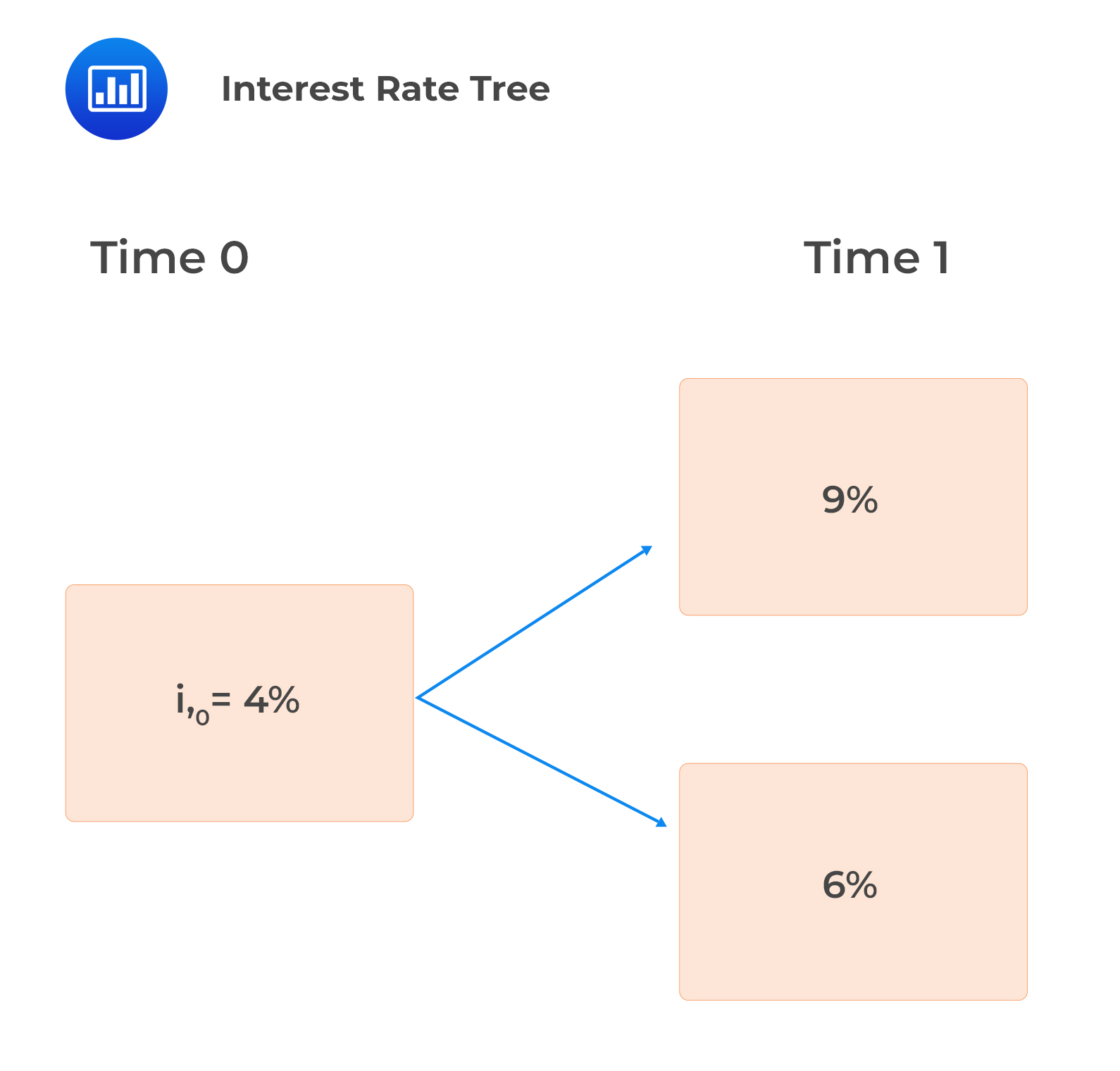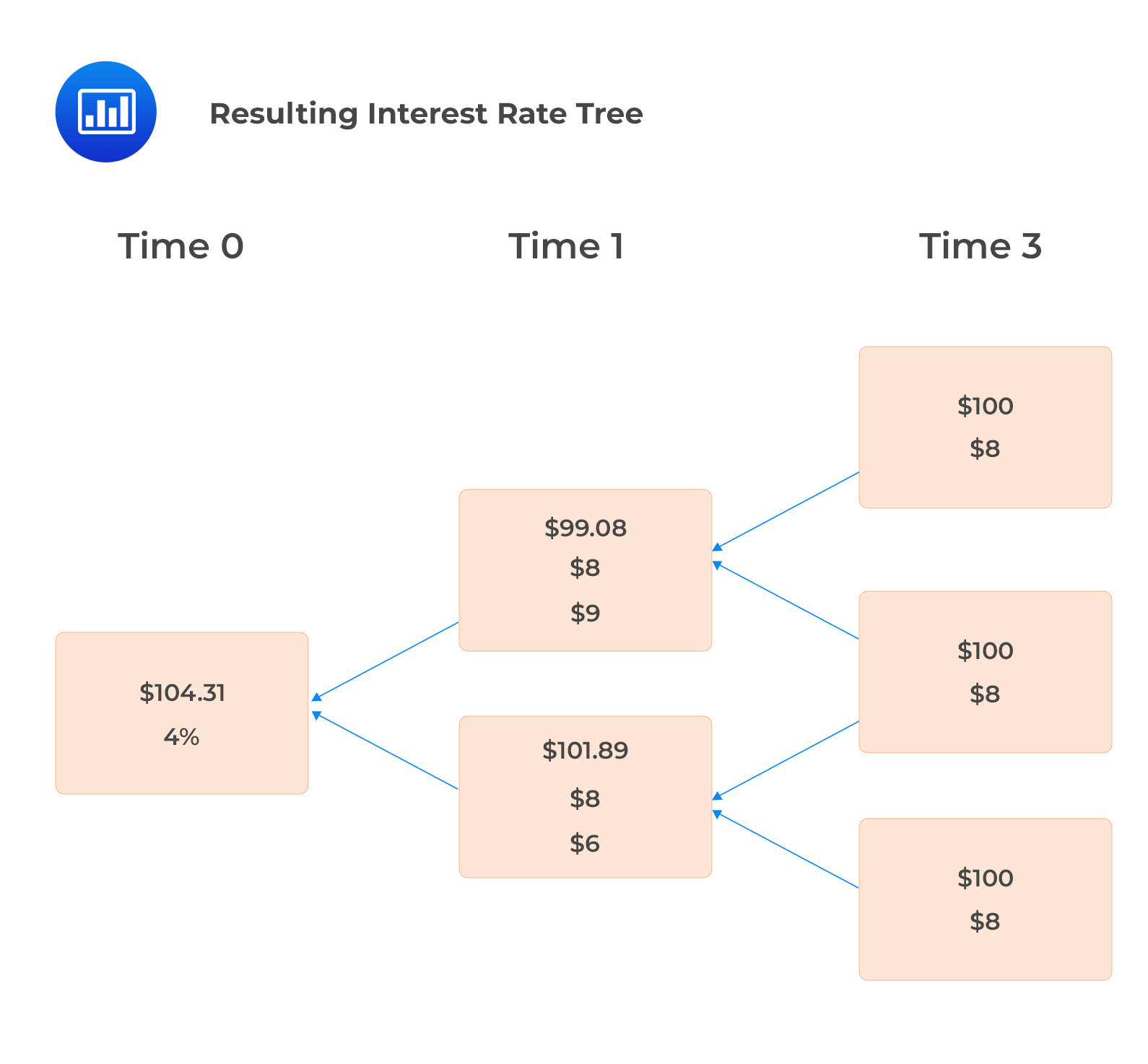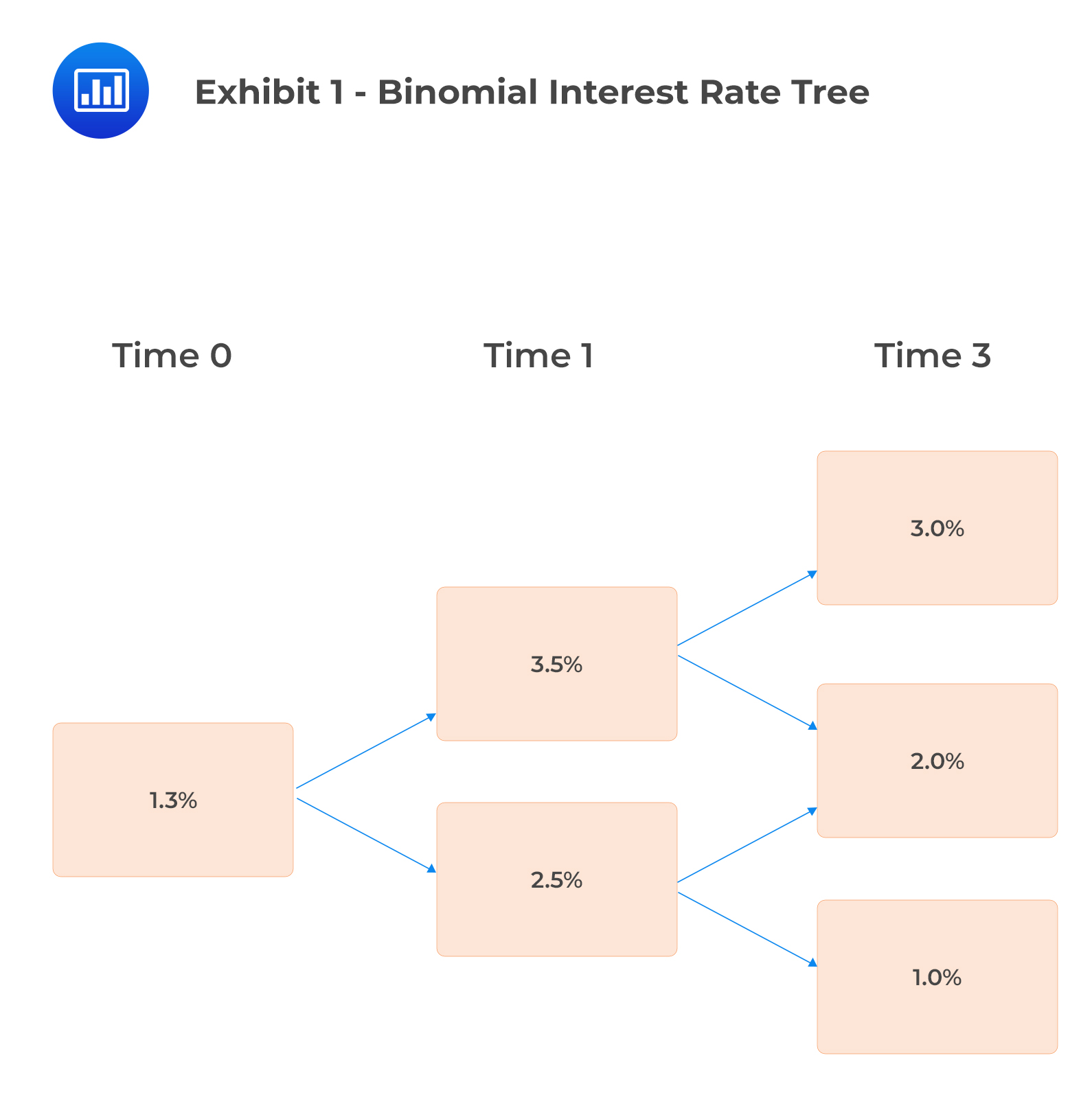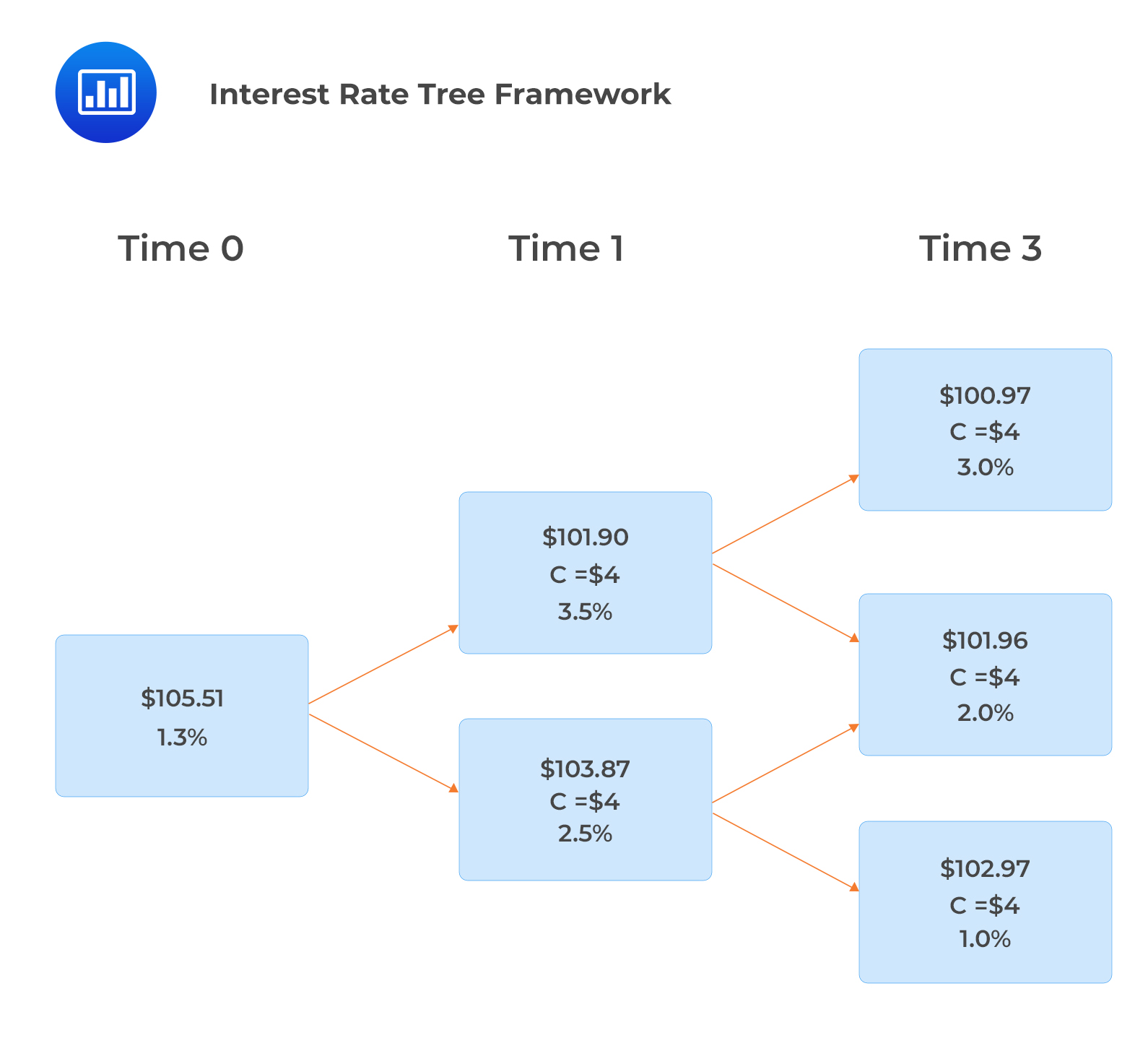# Backward Induction

Backward induction involves working backward from maturity to time 0 to determine a bond’s value at each node. It makes the following assumptions:

1. The value of a bond is known at maturity (Final coupon payment + Face value).
2. The value at each node is the expected present value of two possible values from the next period.
3. The discount rate is the forward rate for that node.

#### Formula: Bond Value at a Node

$$V=0.5\left[\frac{V_u+C}{1+i}+\frac{V_d+C}{1+i}\right]$$

Where:

• $$V_u$$ and $$V_d$$ are the bond values if the higher and lower forward rates, respectively, are realized in one year.
• $$i$$ is the forward interest rate at a particular node.
• $$C$$ is the coupon rate, that is independent of interest rates.

#### Example: Backward Induction

A two-year bond with no embedded options pays 8% annual coupons. Given the following interest rate tree, find the values of the bond at each node, i.e., $$V_{1,u},$$ $$V_{1,d}$$ and $$V_0$$.Solution

\begin{align*} V_{1,u} &=0.5\times\left[\frac{100+8}{1.09}+\frac{100+8}{1.09}\right]=99.08 \\ V_{1,d}& =0.5\times\left[\frac{100+8}{1.06}+\frac{100+8}{1.06}\right]=101.89 \text{ and}\\ V_0 &=0.5\times\left[\frac{99.08+8}{1.04}+\frac{101.89+8}{1.04}\right]=104.31 \end{align*}

The resulting interest rate tree is as shown below:## Question

Exhibit 1 is a binomial interest rate tree related to a bond with annual coupons of 4% with a face value of $100 that matures in three years.Based on Exhibit 1, the price of the bond is closest to: 1.$101.90.
2. $103.87. 3.$105.51.

### Solution

$$V=0.5\left[\frac{V_u+C}{1+i}+\frac{V_d+C}{1+i}\right]$$

The cash flow at Time 3 is 104, the redemption of par value ($100) plus the final coupon payment ($4)

Node values for time two are determined as follows:

\begin{align*} & \text{Upper node: }\frac{104}{1.03}=100.97 \\ & \text{Middle node: }\frac{104}{1.02}= 101.96 \\ & \text{Lower node: }\frac{104}{1.01}= 102.97 \end{align*}

Time 1

\begin{align*} & \text{Upper node: } 0.5\times\left(\frac{100.97+4}{1.035}+\frac{101.96+4}{1.035}\right)= 101.90 \\ & \text{Lower node: } 0.5\times\left(\frac{101.96+4}{1.025}+\frac{102.97+4}{1.025}\right)= 103.87 \end{align*}

Time 0

$$\text{Node value: } 0.5\times\left(\frac{101.90+4}{1.013}+\frac{103.87+4}{1.013}\right)= 105.51$$

Therefore, the price of the bond is \$105.51.

This can be shown in the interest rate tree framework.Reading 29: The Arbitrage-Free Valuation Framework

LOS 29 (e) Describe the backward induction valuation methodology and calculate the value of a fixed-income instrument given its cash flow at each node.

Shop CFA® Exam Prep

Offered by AnalystPrepLevel I
Level II
Level III
All Three Levels
Featured Shop FRM® Exam PrepFRM Part I
FRM Part II
FRM Part I & Part II
Learn with Us

Subscribe to our newsletter and keep up with the latest and greatest tips for success
Shop Actuarial Exams PrepExam P (Probability)
Exam FM (Financial Mathematics)
Exams P & FMGMAT® Complete CourseDaniel Glyn
2021-03-24
I have finished my FRM1 thanks to AnalystPrep. And now using AnalystPrep for my FRM2 preparation. Professor Forjan is brilliant. He gives such good explanations and analogies. And more than anything makes learning fun. A big thank you to Analystprep and Professor Forjan. 5 stars all the way!michael walshe
2021-03-18
Professor James' videos are excellent for understanding the underlying theories behind financial engineering / financial analysis. The AnalystPrep videos were better than any of the others that I searched through on YouTube for providing a clear explanation of some concepts, such as Portfolio theory, CAPM, and Arbitrage Pricing theory. Watching these cleared up many of the unclarities I had in my head. Highly recommended.Nyka Smith
2021-02-18
Every concept is very well explained by Nilay Arun. kudos to you man!2021-02-13